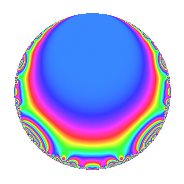# Properties

 Label 1800.1.bk.bLevel 1800 Weight 1 Character orbit 1800.bk Analytic conductor 0.898 Analytic rank 0 Dimension 2 Projective image $$D_{3}$$ CM disc. -8 Inner twists 4

# Related objects

## Newspace parameters

 Level: $$N$$ = $$1800 = 2^{3} \cdot 3^{2} \cdot 5^{2}$$ Weight: $$k$$ = $$1$$ Character orbit: $$[\chi]$$ = 1800.bk (of order $$6$$ and degree $$2$$)

## Newform invariants

 Self dual: No Analytic conductor: $$0.898317022739$$ Analytic rank: $$0$$ Dimension: $$2$$ Coefficient field: $$\Q(\zeta_{6})$$ Coefficient ring: $$\Z[a_1, a_2]$$ Coefficient ring index: $$1$$ Projective image $$D_{3}$$ Projective field Galois closure of 3.1.16200.2 Artin image size $$18$$ Artin image $C_3\times S_3$ Artin field Galois closure of 6.0.25920000.2

## $q$-expansion

The $$q$$-expansion and trace form are shown below.

 $$f(q)$$ $$=$$ $$q + \zeta_{6}^{2} q^{2} + q^{3} -\zeta_{6} q^{4} + \zeta_{6}^{2} q^{6} + q^{8} + q^{9} +O(q^{10})$$ $$q + \zeta_{6}^{2} q^{2} + q^{3} -\zeta_{6} q^{4} + \zeta_{6}^{2} q^{6} + q^{8} + q^{9} + 2 \zeta_{6}^{2} q^{11} -\zeta_{6} q^{12} + \zeta_{6}^{2} q^{16} + 2 q^{17} + \zeta_{6}^{2} q^{18} - q^{19} -2 \zeta_{6} q^{22} + q^{24} + q^{27} -\zeta_{6} q^{32} + 2 \zeta_{6}^{2} q^{33} + 2 \zeta_{6}^{2} q^{34} -\zeta_{6} q^{36} -\zeta_{6}^{2} q^{38} + \zeta_{6} q^{41} -\zeta_{6}^{2} q^{43} + 2 q^{44} + \zeta_{6}^{2} q^{48} -\zeta_{6} q^{49} + 2 q^{51} + \zeta_{6}^{2} q^{54} - q^{57} + \zeta_{6} q^{59} + q^{64} -2 \zeta_{6} q^{66} + \zeta_{6} q^{67} -2 \zeta_{6} q^{68} + q^{72} - q^{73} + \zeta_{6} q^{76} + q^{81} - q^{82} -\zeta_{6}^{2} q^{83} + \zeta_{6} q^{86} + 2 \zeta_{6}^{2} q^{88} - q^{89} -\zeta_{6} q^{96} -\zeta_{6}^{2} q^{97} + q^{98} + 2 \zeta_{6}^{2} q^{99} +O(q^{100})$$ $$\operatorname{Tr}(f)(q)$$ $$=$$ $$2q - q^{2} + 2q^{3} - q^{4} - q^{6} + 2q^{8} + 2q^{9} + O(q^{10})$$ $$2q - q^{2} + 2q^{3} - q^{4} - q^{6} + 2q^{8} + 2q^{9} - 2q^{11} - q^{12} - q^{16} + 4q^{17} - q^{18} - 2q^{19} - 2q^{22} + 2q^{24} + 2q^{27} - q^{32} - 2q^{33} - 2q^{34} - q^{36} + q^{38} + q^{41} + q^{43} + 4q^{44} - q^{48} - q^{49} + 4q^{51} - q^{54} - 2q^{57} + q^{59} + 2q^{64} - 2q^{66} + q^{67} - 2q^{68} + 2q^{72} - 2q^{73} + q^{76} + 2q^{81} - 2q^{82} + q^{83} + q^{86} - 2q^{88} - 2q^{89} - q^{96} + q^{97} + 2q^{98} - 2q^{99} + O(q^{100})$$

## Character Values

We give the values of $$\chi$$ on generators for $$\left(\mathbb{Z}/1800\mathbb{Z}\right)^\times$$.

 $$n$$ $$577$$ $$901$$ $$1001$$ $$1351$$ $$\chi(n)$$ $$1$$ $$-1$$ $$-\zeta_{6}$$ $$-1$$

## Embeddings

For each embedding $$\iota_m$$ of the coefficient field, the values $$\iota_m(a_n)$$ are shown below.

For more information on an embedded modular form you can click on its label.

Label $$\iota_m(\nu)$$ $$a_{2}$$ $$a_{3}$$ $$a_{4}$$ $$a_{5}$$ $$a_{6}$$ $$a_{7}$$ $$a_{8}$$ $$a_{9}$$ $$a_{10}$$
1051.1
 0.5 + 0.866025i 0.5 − 0.866025i
−0.500000 + 0.866025i 1.00000 −0.500000 0.866025i 0 −0.500000 + 0.866025i 0 1.00000 1.00000 0
1651.1 −0.500000 0.866025i 1.00000 −0.500000 + 0.866025i 0 −0.500000 0.866025i 0 1.00000 1.00000 0
 $$n$$: e.g. 2-40 or 990-1000 Significant digits: Format: Complex embeddings Normalized embeddings Satake parameters Satake angles

## Inner twists

Char. orbit Parity Mult. Self Twist Proved
1.a Even 1 trivial yes
8.d Odd 1 CM by $$\Q(\sqrt{-2})$$ yes
9.c Even 1 yes
72.p Odd 1 yes

## Hecke kernels

This newform can be constructed as the intersection of the kernels of the following linear operators acting on $$S_{1}^{\mathrm{new}}(1800, [\chi])$$:

 $$T_{11}^{2} + 2 T_{11} + 4$$ $$T_{17} - 2$$ $$T_{19} + 1$$